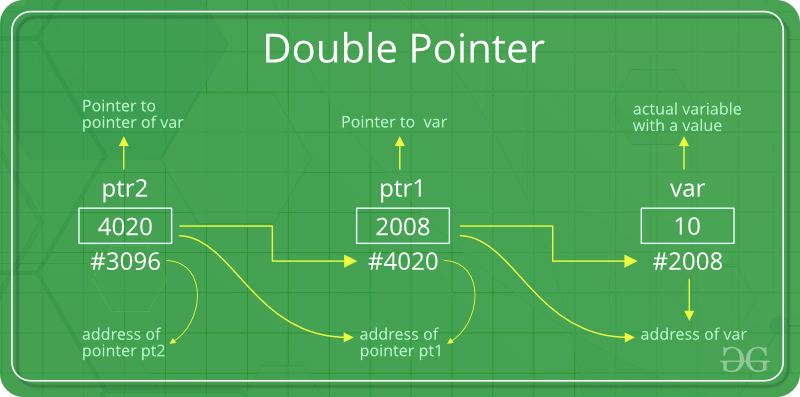# 12.5: Pointers to Pointers

$$\newcommand{\vecs}{\overset { \rightharpoonup} {\mathbf{#1}} }$$ $$\newcommand{\vecd}{\overset{-\!-\!\rightharpoonup}{\vphantom{a}\smash {#1}}}$$$$\newcommand{\id}{\mathrm{id}}$$ $$\newcommand{\Span}{\mathrm{span}}$$ $$\newcommand{\kernel}{\mathrm{null}\,}$$ $$\newcommand{\range}{\mathrm{range}\,}$$ $$\newcommand{\RealPart}{\mathrm{Re}}$$ $$\newcommand{\ImaginaryPart}{\mathrm{Im}}$$ $$\newcommand{\Argument}{\mathrm{Arg}}$$ $$\newcommand{\norm}{\| #1 \|}$$ $$\newcommand{\inner}{\langle #1, #2 \rangle}$$ $$\newcommand{\Span}{\mathrm{span}}$$ $$\newcommand{\id}{\mathrm{id}}$$ $$\newcommand{\Span}{\mathrm{span}}$$ $$\newcommand{\kernel}{\mathrm{null}\,}$$ $$\newcommand{\range}{\mathrm{range}\,}$$ $$\newcommand{\RealPart}{\mathrm{Re}}$$ $$\newcommand{\ImaginaryPart}{\mathrm{Im}}$$ $$\newcommand{\Argument}{\mathrm{Arg}}$$ $$\newcommand{\norm}{\| #1 \|}$$ $$\newcommand{\inner}{\langle #1, #2 \rangle}$$ $$\newcommand{\Span}{\mathrm{span}}$$

# Double Pointer (Pointer to Pointer) in C

We already know that a pointer points to a location in memory and thus used to store the address of variables. So, when we define a pointer to pointer. The first pointer is used to store the address of the variable. And the second pointer is used to store the address of the first pointer. That is why they are also known as double pointers.

How to declare a pointer to pointer in C++?
Declaring Pointer to Pointer is similar to declaring pointer in C. The difference is we have to place an additional ‘*’ before the name of pointer.
Syntax:

int **ptr2;   // declaring double pointers
int *ptr1;    // pointer
int var;      // variable


Below diagram explains the concept of Double Pointers:The above diagram shows the memory representation of a pointer to pointer. The first pointer ptr1 stores the address of the variable and the second pointer ptr2 stores the address of the first pointer.

Remember how the pointer works - we saw the next image in a previous section...just for a reminder.

Let us understand this more clearly with the help of the program below:

##include <iostream>
using namespace std;

// C program to demonstrate pointer to pointer
int main()
{
int newVar = 789;
// pointer for newVar
int *newPtr;
// double pointer for newPtr
int **dblPtr;

// storing address of newVar in newPtr
newPtr = &newVar;

// Storing address of newPtr in dblPtr
dblPtr = &newPtr;

// Displaying address of all the varaibles
cout << "Address of newVar: " << &newVar << endl;
cout << "Address contained in newPtr: " << newPtr << endl;
cout << "Address contained in dblPtr: " << *dblPtr << endl;

// Displaying value of newVar using
// both single and double pointers
cout << "Value of newVar = " << newVar << endl;
cout << "Value of newVar using single pointer, *newPtr = " << *newPtr << endl;
cout << "Value of newVar using double pointer, **dblPtr = " << **dblPtr << endl;

return 0;
} 

We have an integer variable, a pointer variable and a pointer to a pointer variable. Really, all we are doing is passing around the address of the integer variable, As you can see in the output below, the address is the same, and accessing the value is gives us that same value..

Here is the output:

Address of newVar: 0x7ffe33b7c834
Value of newVar using double pointer, **dblPtr = 789